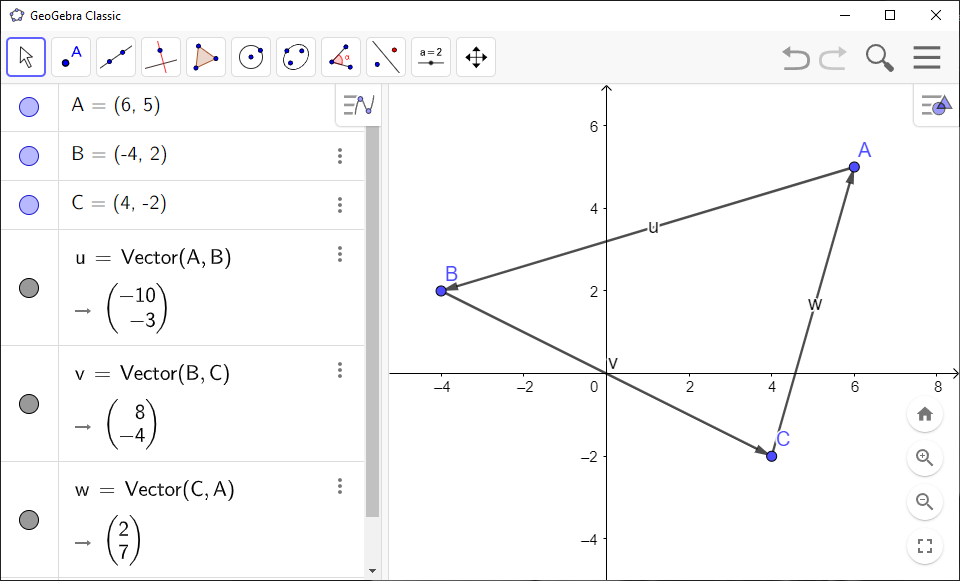# How to Graph Vector Sums and Differences in GeoGebra

You can use `GeoGebra` to draw the sum and difference of vectors:

`GeoGebra` Instruction 1

1.
Open `Algebra View` and `Graphics View` under`View` in`Menu`.
2.
Create three points $A$, $B$ and $C$.
3.
Use `Vector`to make the vector from $A$ to $B$, the vector from $B$ to $C$, and the vector from $A$ to $C$.

You can see from the figure you have made that $\stackrel{\to }{AB}+\stackrel{\to }{BC}=\stackrel{\to }{AC}$. You can also see that $\stackrel{\to }{AC}-\stackrel{\to }{AB}=\stackrel{\to }{BC}$.